## EMAP2SEC+

Emap2sec+ is a computational tool using deep learning that can accurately identify structures, alpha helices, beta sheets, other(coils/turns) and DNA/RNA, in cryo-Electron Microscopy (EM) maps of medium to low resolution.

## Introduction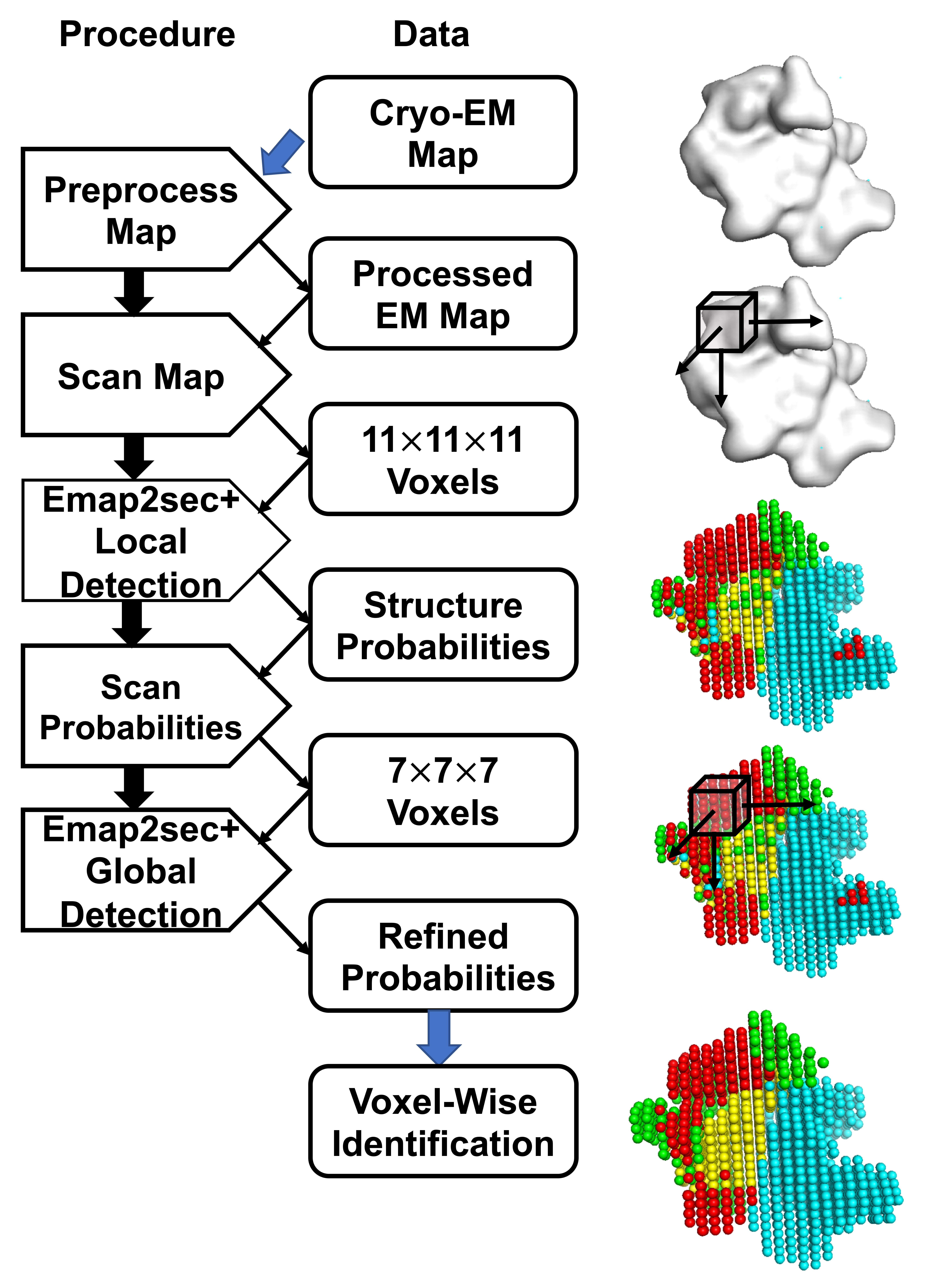# Architecture

### This document will provide a detailed explanation of each step of the Emap2sec+ architecture and programs needed to run those steps. It concludes by giving a step-by-step application walk-through on a simulated and an experimental EM map.### Different EM maps have different grid size. Considering that we use density values in grid points as our model’s input, we need to make them to have the same measurement across different maps. An automatic process will change the grid size of input map to 1 Å.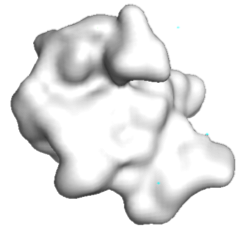### The input file for Emap2sec+ is generated in two-steps and automatically finished in backend. In the first step, a program named map2train will take an EM map and map's author recommended contour level as input and generates an intermediate readable text file called [map_name].trimmap. A trimmap contains normalized electron density values of voxels. In the second step, a process will use the trimmap file to generate input file (*.input) for Emap2sec+ model, which contains density values by scanning the input map in all the 3 directions using a 11*11*11 cube with stride=2. Emap2sec+ makes structure assignment to each of these rows.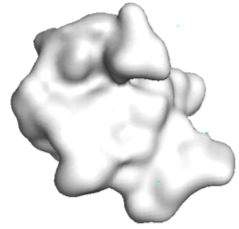### In the figure, red spheres correspond to helix predictions, yellow spheres correspond to sheet predictions, green spheres correspond to other (coil/turn) predictions and cyan spheres correspond to DNA/RNA predictions.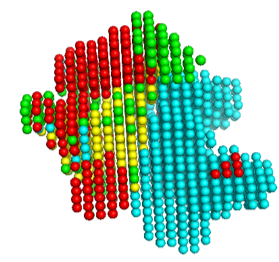### The output from the first phase is a set of predicted probabilities for each of helix, sheet, other protein structures and DNA/RNA. In this second phase, these probabilities are used to further augment predictions. The final voxel-wise structure prediction for the cryo-EM map is done using the output labels from phase 2." to the end of section 4.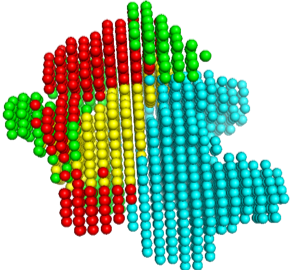# Simulated map example (10Å resolution)

`python3 main.py --mode=0 -F=[Map_path] --type=1 --gpu=0 --class=4 `

### The example input map is included in 5T5K .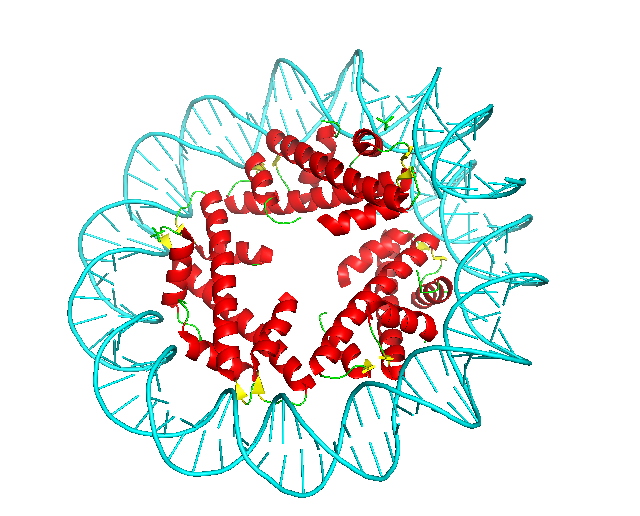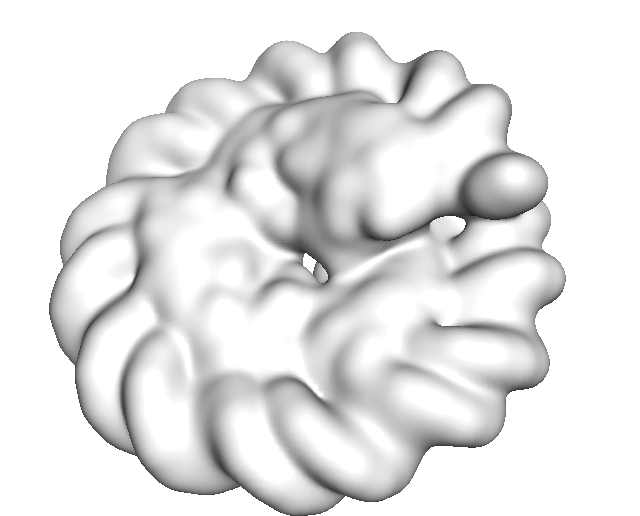### Results are saved in Predict_Result/SIMU10/[Input_Map_Name]. Phase 1 and Phase 2 visualization results (Pymol sessions) are saved in “Phase1” and “Phase2” sub-directory, respectively. You will find generated *.pml files generated to visualize. Please use “pymol -u *.pml” to visualize the final structure detection result. If you want to only see very confident detection results, run “pymol -u *C.pml” using another visualization file named "*C.pml", which only includes confident detections with a probability>=0.9.

`pymol -u *.pml`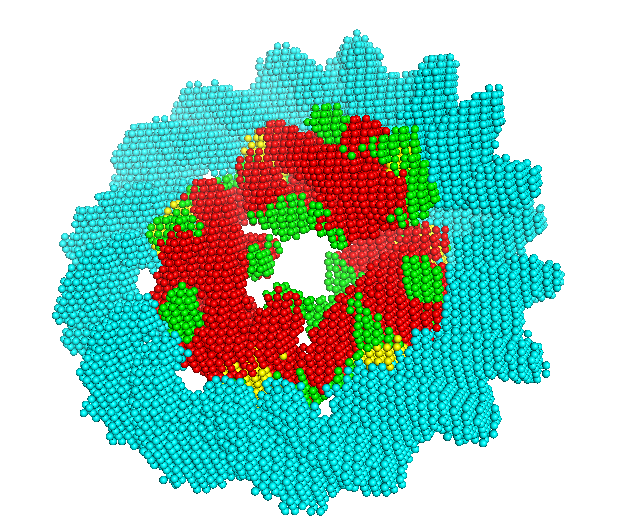### In the case that you are testing the software with a case, you can check the accuracy of the structure detection by Emap2sec+ by comparing the result with the known structure. This mode cannot be used in real scenarios where the native structure is not available. We usually use the mode to evaluate Emap2sec+ performance on testing dataset with known structures to verify its performance. This mode is also useful to measure the difference of the detected structure by Emap2sec+ with the structure currently assigned to the EM map. `python3 main.py --mode=1 -F=[Map_path] ---P=[PDB_Path] type=1 --gpu=0 --class=4` In the Predict_Result_WithPDB/SIMU10/[Input_Map_Name], our evaluation report will be saved in *_report.txt. Here is an example of our evaluation report of 5T5K.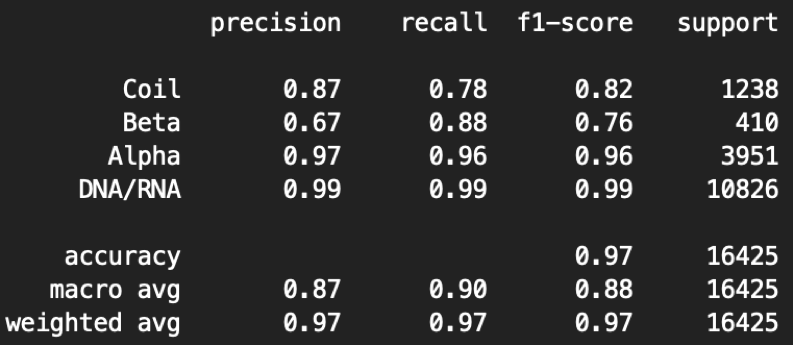Here the precision is the fraction of correct predicted structures among the specific predicted structure, while recall (also known as sensitivity) is the fraction of the total amount of the specific structure that were actually retrieved. The F1 score is the harmonic mean of the precision and recall. The support is the number of voxels with the structure label. The macro measurement means macro-averaging(taking all classes as equally important), while the micro means mirco-averaging (biased by class frequency).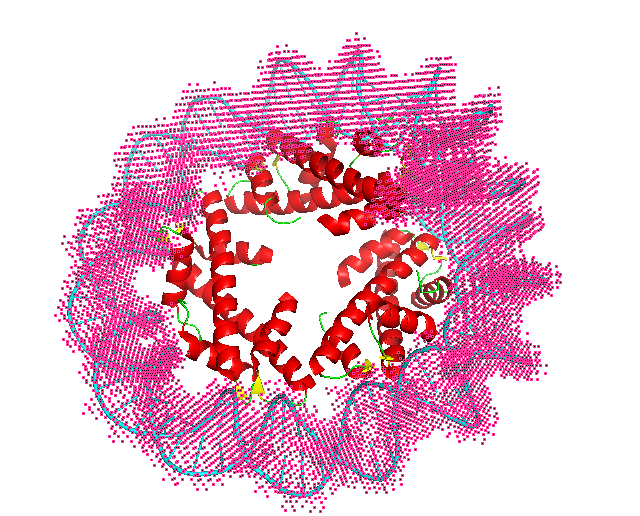# Experimental map example

### `python3 main.py --mode=0 -F=[Map_path] –type=3 --gpu=0 --class=4 --fold=3 -–contour=0.006` If the map grid size is smaller than 1, you also need to specify –-resize=1 to resize the grid size in the command line. This will make the command run slower than the default mode. The example input map is included in 6BJS, which is in the fold 3 testing dataset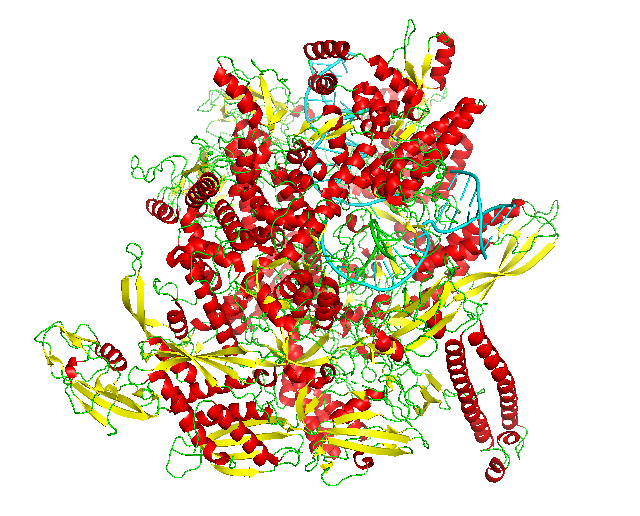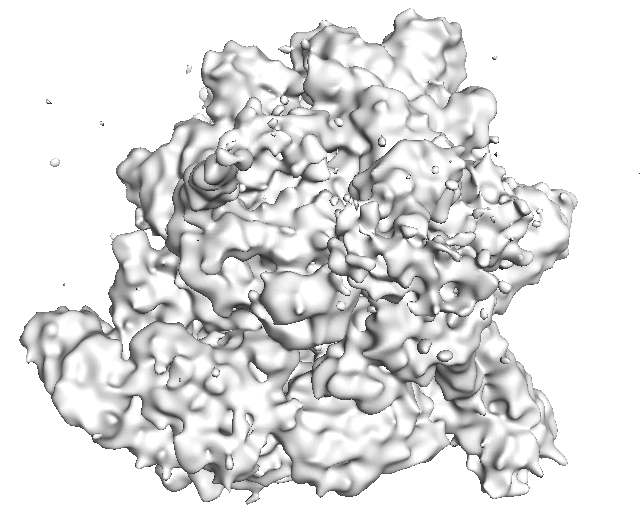### Results are saved in Predict_Result/REAL/Fold3_Model_Result/[Input_Map_Name]. Phase 1 and Phase 2 visualization results (Pymol sessions) are saved in “Phase1” and “Phase2” sub-directory, respectively. You will find generated *.pml files generated to visualize. Please use “pymol -u *.pml” to visualize the final structure detection result. If you want to only see very confident detection results, run “pymol -u *C.pml” using another visualization file named "*C.pml", which only includes confident detections with a probability>=0.9.

`pymol -u *.pml`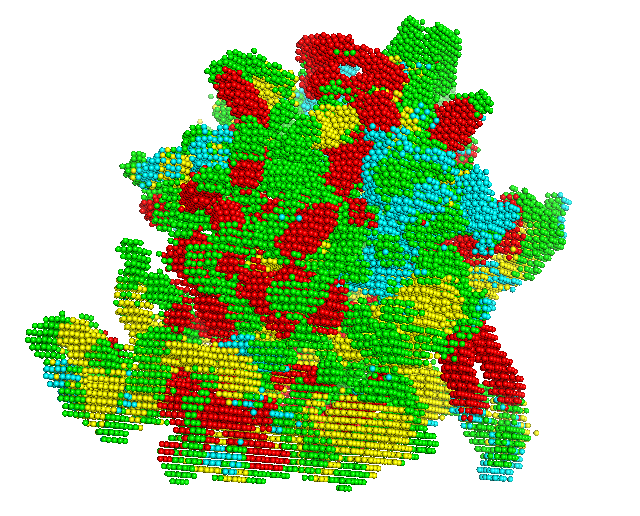### 3 Evaluate Performance(only when the correct underlined structure in the map is known)

In the case that you are testing the software with a case, you can check the accuracy of the structure detection by Emap2sec+ by comparing the result with the known structure. This mode cannot be used in real scenarios where the native structure is not available. We usually use the mode to evaluate Emap2sec+ performance on testing dataset with known structures to verify its performance. This mode is also useful to measure the difference of the detected structure by Emap2sec+ with the structure currently assigned to the EM map.

### `python3 main.py --mode=1 -F=[Map_path] ---P=[PDB_Path] --type=3 --gpu=0 --class=4 -–fold=3 -–contour=0.006` In the Predict_Result_WithPDB/REAL/Fold3_Model_Result/[Input_Map_Name], our evaluation report will be saved in *_report.txt. Here is an example of our evaluation report of 6BJS.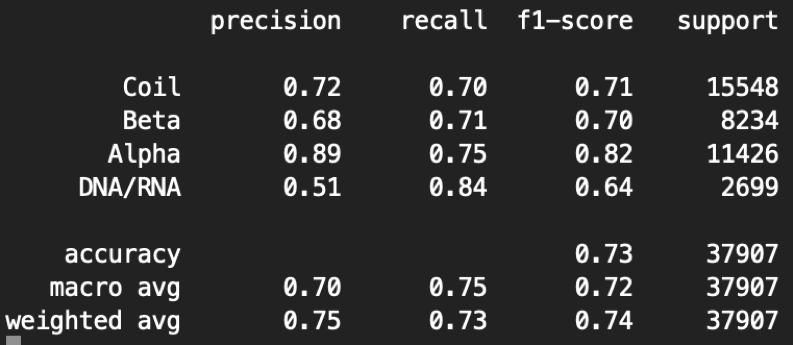Here the precision is the fraction of correct predicted structures among the specific predicted structure, while recall (also known as sensitivity) is the fraction of the total amount of the specific structure that were actually retrieved. The F1 score is the harmonic mean of the precision and recall. The support is the number of voxels with the structure label. The macro measurement means macro-averaging(taking all classes as equally important), while the micro means mirco-averaging (biased by class frequency).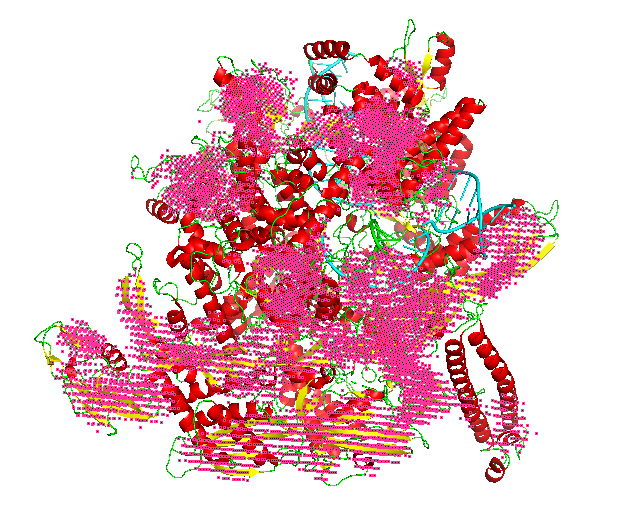# Availability

Recommended:: You can run Emap2sec+ on Goole Colab.
You can also run Emap2sec+ on CodeOcean.
Full codes of Emap2sec+ are available at GitHub.